#Quality Digest

Featured Product
This Week in Quality Digest Live
Six Sigma Features
Ken Maynard
How to create a continuous improvement culture in any organization
Jody Muelaner
A standard set of graphical methods for improving quality
Dirk Dusharme @ Quality Digest
Making better use of taxpayer dollars
Ryan E. Day
Case studies in saving taxpayer dollars with lean Six Sigma
Taran March @ Quality Digest
Lean Six Sigma has arrived on a few campuses. Will more follow?
Six Sigma News
Floor symbols and decals create a SMART floor environment, adding visual organization to any environment
A guide for practitioners and managers
Making lean Six Sigma easier and adaptable to current workplaces
Gain visibility into real-time quality data to improve manufacturing process efficiency, quality, and profits
Makes it faster and easier to find and return tools to their proper places
Version 3.1 increases flexibility and ease of use with expanded data formatting features
Provides accurate visual representations of the plan-do-study-act cycle
SQCpack and GAGEpack offer a comprehensive approach to improving product quality and consistency
Customized visual dashboards by Visual Workplace help measure performanceBio

Six Sigma

## Why Use Ranges?

### What they didn’t teach in your stat class

Published: Monday, February 3, 2014 - 17:29

Last month in “The Analysis of Experimental Data,” I presented a method for analyzing experimental data that was built on the use of the range statistic as a measure of dispersion. In this day of computers and software, why should we even consider using ranges in our analysis of experimental data? Wouldn’t other, more efficient measures of dispersion do a better job? Since these questions can be a barrier to the effective analysis of data, they deserve to be answered.

Last month I showed how to use the analysis of means (ANOM). When Ellis Ott introduced this technique in 1967, he used the average range as the basis for obtaining the ANOM detection limits. When Ed Schilling extended Ott’s technique in 1973, he also used the average range as the basis for obtaining the ANOM detection limits. In these early papers the scaling factors given were merely upper bounds on the exact values. In 1974 Lloyd Nelson recomputed these upper bounds to obtain more precise ANOM detection limits. However, in his work Nelson shifted from using the average range to using a different dispersion statistic. He used the root mean square within (RMSW). We will return to this in a moment.

In 1975 Ott used the average of upper and lower bounds on the scaling factors to obtain sharper more precise ANOM detection limits based on the average range statistic. Finally, in 1982 Peter Nelson computed exact critical values for ANOM based on the RMSW statistic. At my suggestion, Peter extended these tables in 1993. Thus, from the beginning, much effort was spent in refining and obtaining exact scaling factors for ANOM. Along the way a shift occurred in the dispersion statistic used in the computation. Because this shift is not a trivial shift and has some unintended consequences, we need to compare the use of these two measures of dispersion.

### The root mean square within statistic

For more than 80 years the mean square within (MSW) has been the standard yardstick for characterizing the within-subgroup dispersion in the analysis of variance (ANOVA). Given k subgroups of size n, the MSW is simply the average of the k subgroup variance statistics: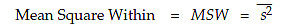The reason that the MSW works so well with ANOVA is due to the nature of the ANOVA technique. In ANOVA we essentially compute a signal-to-noise ratio. While the MSW provides the most efficient unbiased estimate of the noise component, the signal component is found by squaring all the potential signals and adding them up. ANOVA then compares the average of the squared signals with the MSW. This computation of a signal-to-noise ratio has been found to be very robust and satisfactory in more than 80 years of use.

Because of this success, it is almost natural for statisticians, who are extensively trained in ANOVA techniques, to immediately think of using the RMSW for applications requiring an estimate of the standard deviation parameter.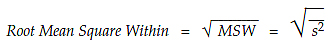The mean square within is completely appropriate for ANOVA, however, the RMSW has a drawback that makes it inappropriate for ANOM: The MSW is robust when it is used with ANOVA, but the RMSW suffers from a lack of robustness.

### Robustness

The comparison made in ANOM is different from the comparison made in ANOVA. In ANOM the potential signals are simply plotted one by one, and those that cross over the detection limit are those that are likely to be real. Thus, in ANOM we filter out the probable noise by the computation of the detection limit. And as you can see from the brief history given above, much effort has been put into the problem of sharpening up the computation of these detection limits.

So, why not use the RMSW to compute ANOM limits? Because the RMSW is not robust. When the subgroups do not all display the same amount of variation, the RMSW will be more inflated than will the average range. This will result in limits that are wider than they should be, which will make the analysis less sensitive than intended.

To illustrate this problem I will use an example from one of my clients. These data consist of ten subgroups of size two. The Averages, Ranges, and Variances for each sub­group are listed in figure 1.Figure 1: The Gauge Study Data

Even a cursory glance will show that the last subgroup has a different amount of variation from the others. To see the impact of this subgroup we shall compute measures of dispersion using the first nine subgroups and then using all ten subgroups.

The first nine subgroups have an average range of 3.333. Yet the average range for all ten subgroups is 5.5. Thus, the excessive variation in the last subgroup inflates the average range by 65%.

The first nine subgroups have an RMSW of 2.89. Yet the RMSW for all ten subgroups is 6.22. Thus, the last subgroup inflated the RMSW by 115%, which is almost twice the inflation shown by the average range. This tendency toward excess inflation is inherent in the RMSW. By squaring the standard deviations, averaging them, and then finding the square root, the RMSW automatically gives more weight to the larger values.

Thus, the RMSW is not robust to differences in variation. This lack of robustness is not so much of a problem with ANOVA as it is with ANOM. This is because in ANOVA we are squaring the signals as well as squaring the noise. (When the signals are larger than the noise, their squares increase even more rapidly than the contamination in the MSW.) However, when we are computing limits to use with the raw signals themselves this lack of robustness can result in detection limits that are more inflated than those found using the average range. It is this lack of robustness that makes the RMSW an inappropriate measure for use with ANOM or process behavior charts.

This subtle point was missed by those that shifted to using the RMSW with ANOM. While they were busy refining the upper bounds provided by Ott in order to sharpen up the detection limits, their shift to using the RMSW ignored its lack of robustness. The use of the average range provides additional robust­ness to the analysis that is missing when the RMSW is used. Figure 2 shows the ANOM plots for figure 1 with detection limits based on each measure of dispersion.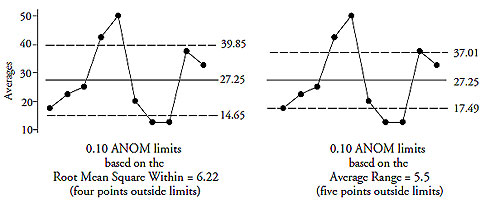Figure 2: The average range is more robust than the root mean square within

So, you paid good money to conduct the experiment and have collected your data, does it make sense to use an analysis that will miss some of the signals you have paid to discover?

### Equivalence

“Okay, the root mean square within is not robust for ANOM. But I was taught that the range is inefficient, so what about using the standard deviations instead of the variances?”

It is true that the range is an inefficient summary for a large data set, but this does not present a problem for ANOM and process behavior charts because they are built on using small sets of data. However, let us consider what would happen if we were to use the subgroup standard deviations instead of the ranges. If we return to the data of figure 1 and consider the subgroup standard deviations, we have the values shown in figure 3.

The first nine subgroups have an average standard deviation of 2.36. Yet the average standard deviation for all 10 subgroups is 3.89. Thus, the excessive variation in the last subgroup inflates the average standard deviation by 65 percent. This is exactly the same as the inflation seen with the average range described in the previous section.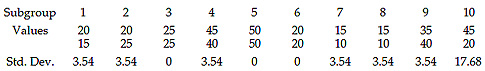Figure 3: Standard deviations for the gauge study data

As I showed in my book Advanced Topics in Statistical Process Control (SPC Press, 2004), the range is essentially equivalent to the standard deviation statistic when the subgroup size is smaller than 15. The graphs shown in figure 4 superimpose the distributions of both statistics after adjusting for their different biases. In the case of n = 2 there is only one curve because of the deterministic relationship between the range and the standard deviation. For the larger values of n there are two curves shown. After adjusting each distribution for the average bias of the statistic we end up with curves that are so close together that it’s an eye test to separate them. In each case the differences between the two distributions shown for each value of n in figure 4 are too small to be of any practical interest.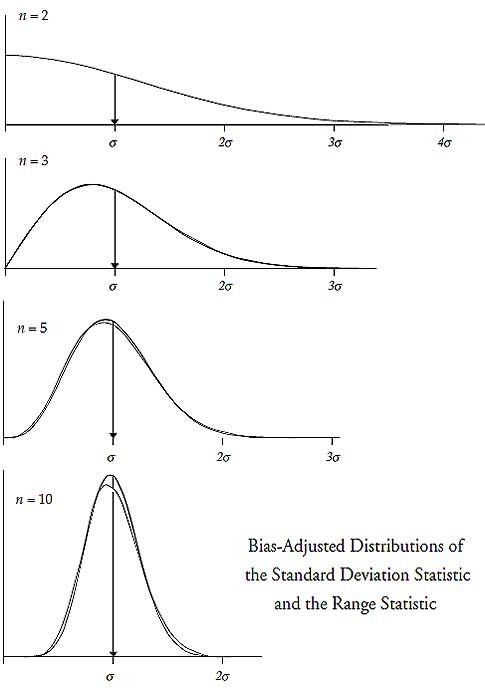Figure 4: The range and standard deviation are equivalent for small n

Not only are the distributions essentially the same, but the individual statistics for a given subgroup are highly correlated with each other when n is less than 15. Figure 5 shows the correlations between the bias-adjusted ranges (on the x-axis) and the bias-adjusted standard deviation statistics (on the y-axis) for each of four sets of 100 subgroups.Figure 5: Correlations between ranges and standard deviations

The correlation is 1.00 when n = 2 and it is 0.996 when n = 3. Then, as n increases from 3 to 15 the correlation between the range and the standard deviation drops approximately 0.01 with each unit change in n. Thus, as long as your subgroup size is less than 12, the range and the standard deviation will have a correlation greater than 90 percent.

The fact that these statistics are equivalent for small subgroup sizes means that the choice between using a range and using a standard deviation statistic to measure the variation within the subgroups is merely a personal preference rather than being a matter of mathematical efficiency. Some people actually prefer complexity. Regardless of your personal preference, both the average standard deviation and the average range will work equally well with small subgroups. However, the average range has one advantage that the average standard deviation lacks.

### Transparency

On a completely different level there is another reason to use the range. For more than 40 years I have had the job of teaching statistical techniques to nonmathematicians. As part of this job I have had the opportunity to look in into student’s faces as explanations are given and I have discovered that even among those who know how to compute a standard deviation statistic there is less resistance when I use a range. A range is an intuitive and transparent measure of dispersion. The standard deviation statistic is neither.

For example, I find that people seem to understand the average as the center of mass for the data. I suspect that this is because balance is one of those things we learn as a toddler. Yet when I extend this physical analogy and explain the variance statistic as the rotational inertia for the data, I tend to get blank looks. I suspect that this is because we are not ordinarily concerned with rotational inertia except on those occasions when we slip on the ice and lose our balance.

So, whenever I use the range in my analysis I find that the explanation is generally met with understanding and acceptance. On the other hand, when I use the standard deviation in my analysis, I find that the explanation gets derailed. As soon as I mention the standard deviation, half the class gets a blank look in their eyes and the other half starts trying to figure out how the standard deviation fits into the analysis. Either way, the explanation has been derailed and turns into gobbledygook. Since the most important part of any analysis is the communication of the results, it is important to avoid gobbledygook, and one of the easiest ways to do this is to avoid using the standard deviation statistic. After all, even if someone does understand the concept of rotational inertia, they are still unlikely to understand exactly what the square root of rotational inertia represents.

### Summary

Thus, there are three reasons I use the average range with ANOM and process behavior charts rather than the RMSW. The first of these is mathematical robustness; the second is the practical equivalence of the range and the standard deviation statistic for small subgroup sizes; and the third is the intuitive and transparent nature of the range. In keeping with the principle that the best analysis is the simplest analysis that allows you to discover the signals contained within your data, the range-based ANOM is not only robust, intuitive, transparent, and easy—it’s also hard to beat.

Donald J. Wheeler is a Quality Digest content partner.

### Discuss### Donald J. Wheeler

Dr. Donald J. Wheeler is a Fellow of both the American Statistical Association and the American Society for Quality, and is the recipient of the 2010 Deming Medal. As the author of 25 books and hundreds of articles, he is one of the leading authorities on statistical process control and applied data analysis. Find out more about Dr. Wheeler’s books at www.spcpress.com.

Dr. Wheeler welcomes your questions. You can contact him at djwheeler@spcpress.com